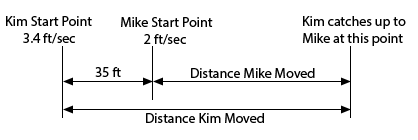Paul's Online Notes
Home / Algebra / Solving Equations and Inequalities / Applications of Linear Equations
Show Mobile Notice Show All Notes Hide All Notes
Mobile Notice
You appear to be on a device with a "narrow" screen width (i.e. you are probably on a mobile phone). Due to the nature of the mathematics on this site it is best views in landscape mode. If your device is not in landscape mode many of the equations will run off the side of your device (should be able to scroll to see them) and some of the menu items will be cut off due to the narrow screen width.

### Section 2.3 : Applications of Linear Equations

4. Mike starts out 35 feet in front of Kim and they both start moving towards the right at the same time. Mike moves at 2 ft/sec while Kim moves at 3.4 ft/sec. How long will it take for Kim to catch up with Mike?

Show All Steps Hide All Steps

Start Solution

Let’s start with a diagram of what is going on in this situation.Show Step 2

We can next set up a word equation for this situation.

$\left( \begin{array}{c}{\mbox{Distance}}\\ {\mbox{Kim Moved}}\end{array} \right) = 35 + \left( \begin{array}{c}{\mbox{Distance}}\\ {\mbox{Mike Moved}}\end{array} \right)$

We know that Distance = Rate X Time so this gives to following word equation.

$\left( \begin{array}{c}{\mbox{Rate of}}\\ {\mbox{Kim}}\end{array} \right)\left( \begin{array}{c}{\mbox{Time of}}\\ {\mbox{Kim}}\end{array} \right) = 35 + \left( \begin{array}{c}{\mbox{Rate of}}\\ {\mbox{Mike}}\end{array} \right)\left( \begin{array}{c}{\mbox{Time of}}\\ {\mbox{Mike}}\end{array} \right)$ Show Step 3

Both Kim and Mike move for the same amount of time so let’s call that $$t$$. We also know that Kim moves at 3.4 ft/sec while Mike moves at 2 ft/sec. Plugging all this information into the word equation above gives the following equation.

\begin{align*}\left( {3.4} \right)\left( t \right) & = 35 + \left( 2 \right)\left( t \right)\\ 3.4t & = 35 + 2t\end{align*} Show Step 4

Now we can solve this equation for the time they traveled.

\begin{align*}3.4t & = 35 + 2t\\ 1.4t & = 35\\ t & = 25\end{align*}

So, Kim will catch up with Mike after she moves for 25 seconds.